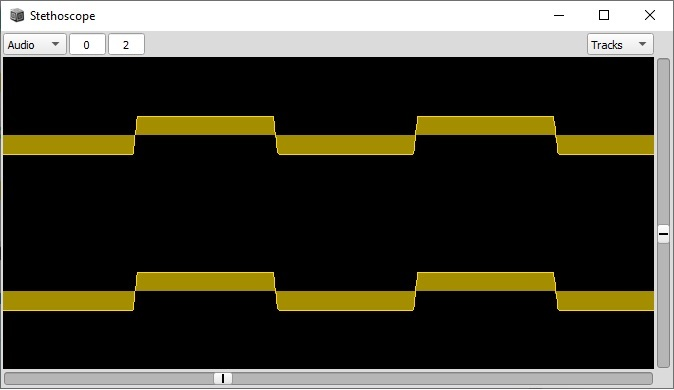# SinOsc into clipper into sine function

I am trying to covert some reaktor code to supercollider
A sineosc ( voume exceeds 1 ) into clipper =0.5,-0.5 for clipp values into a sine fucntion
See screenshot and waveform
In supercollider it sems that the sine function behaves differently , even when I mulitply the clipped output befor going into the sie function , the result i still a full legth cycle , in contrast with reaktor

(
{

``````	var a,b,c,d;
a=SinOsc.ar([100,100])*10;//Loud sinewave
b=Clip.ar(a,-0.5,0.5)*1;//clip values
c=(b*1).sin;//multiply befor going into sine funcion
d=c*0.5///global voume scaling
}.
play
)
``````What is the input range of Sine/Cosine in Reaktor?

I’ll take a guess that it might be +/- 1 (so that you’d get a complete cycle with saw LFO ranging between -1 to +1). Pure Data’s cos~ object does something similar.

`sin()` in SC expects radians (so the complete cycle would be -pi to +pi).

Maybe try scaling the `sin()` input by pi? `c = (b * pi).sin;` – or maybe `b * 2pi` to compensate for the clip to +/-0.5.

hjh

Yes input range off the sine in reaktor is 1 , which equals to 0dbfs , but it’s multiplied when going into the mixer , substitue in supercollider is *10 ( I even tried multiple factors )

Scaling it by pi is the same as multiply with 3.1415
(bpi) , same as (b3.1415…)
Anyway , it don’t get the same result …
Willt try further

You’re multiplying by 10 and then clipping to +/-0.5.

So the output range of the clipped signal is +/-0.5. “But it’s multiplied” is irrelevant.

Now… if 0.5 in Reaktor is half a sine cycle, this will be pi in standard math. So my initial guess was wrong – you would need a factor that would map the 0.5 clipped value onto pi… so `sin(b * 2pi)`.

I’m still not totally sure if Reaktor does a full cycle for -1 to +1, or 0 to 1. If the former, my “pi” guess might have been right. Since that wasn’t right, it’s likely to be the latter (–> 2pi).

hjh